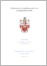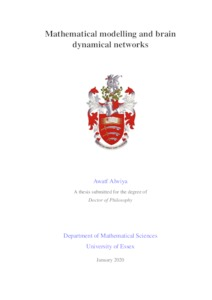# Mathematical modelling and brain dynamical networks

Alwiya, Awatf (2020) Mathematical modelling and brain dynamical networks. PhD thesis, University of Essex.Preview
Text
Thesis-BDNs 2020.pdf

Download (7MB) | Preview

## Abstract

In this thesis, we study the dynamics of the Hindmarsh-Rose (HR) model which studies the spike-bursting behaviour of the membrane potential of a single neuron. We study the stability of the HR system and compute its Lyapunov exponents (LEs). We consider coupled general sections of the HR system to create an undirected brain dynamical network (BDN) of Nn neurons. Then, we study the concepts of upper bound of mutual information rate (MIR) and synchronisation measure and their dependence on the values of electrical and chemical couplings. We analyse the dynamics of neurons in various regions of parameter space plots for two elementary examples of 3 neurons with two different types of electrical and chemical couplings. We plot the upper bound Ic and the order parameter rho (the measure of synchronisation) and the two largest Lyapunov exponents LE1 and LE2 versus the chemical coupling gn and electrical coupling gl. We show that, even for small number of neurons, the dynamics of the system depends on the number of neurons and the type of coupling strength between them. Finally, we evolve a network of Hindmarsh-Rose neurons by increasing the entropy of the system. In particular, we choose the Kolmogorov-Sinai entropy: HKS (Pesin identity) as the evolution rule. First, we compute the HKS for a network of 4 HR neurons connected simultaneously by two undirected electrical and two undirected chemical links. We get different entropies with the use of different values for both the chemical and electrical couplings. If the entropy of the system is positive, the dynamics of the system is chaotic and if it is close to zero, the trajectory of the system converges to one of the fixed points and loses energy. Then, we evolve a network of 6 clusters of 10 neurons each. Neurons in each cluster are connected only by electrical links and their connections form small-world networks. The six clusters connect to each other only by chemical links. We compare between the combined effect of chemical and electrical couplings with the two concepts, the information flow capacity Ic and HKS in evolving the BDNs and show results that the brain networks might evolve based on the principle of the maximisation of their entropies.

Item Type: Thesis (PhD) Q Science > QA Mathematics Faculty of Science and Health > Mathematical Sciences, Department of Awatf Alwiya 02 Jul 2020 16:12 02 Jul 2020 16:12 http://repository.essex.ac.uk/id/eprint/28016

### Actions (login required)View Item